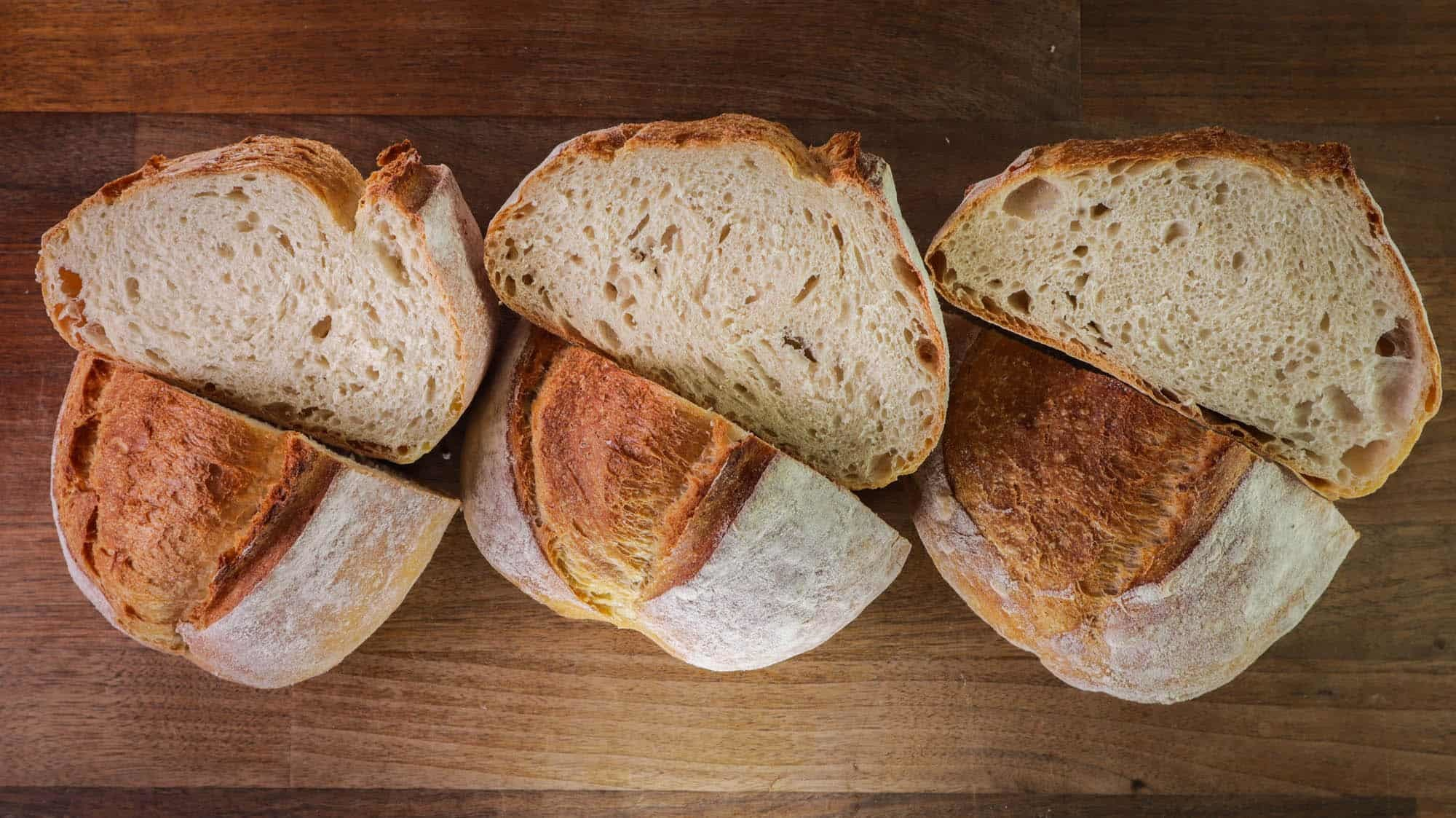# Baker’s Percentage And Dough Hydration Explained9 9 Baker’s Percentage And Dough Hydration Explained

Baker’s percentage or baker’s maths is a great tool for creating your own recipes. Understanding dough hydration is one of the fundamentals of baking.

Baker’s percentage is a way of calculating the amount of any ingredient in relation to the total flour in the recipe.

No matter how much flour is being used, from 250g to make a small loaf at home to 2 tons of flour in a bread factory, the flour will always be 100%.

Every other ingredient is calculated as a percentage of the total amount of flour.

Hydration is the amount of water in dough. It can range from as little as 50% to more than 100%. I imagine you can kind of start seeing the point here. If the flour is always 100%, and the water is, for example 50%, then the amount of water will be equal to half of the amount of flour.

Example. A dough is made of 500g flour. I want it to be 60% hydration because that is a regular amount which will get me a nice loaf. To work out the amount of water simply calculate 60% from 500g.

500 x 0.6 = 300g

This goes for any ingredient.

Understanding these simple calculations opens a whole world of baking. You no longer have to follow recipes. You can create your own recipes and be in full control every single time!

Watch the video down below where I explain everything in detail.

Watch the video here